| Next|| Previous || Top |

# EXCHANGE_SPECIES

This keyword data block is used to define a half-reaction and relative log K for each exchange species. Normally, this data block is included in the database file and only additions and modifications are included in the input file.

### Example data block

```Line 0:  EXCHANGE_SPECIES
Line 1a:      X- = X-
Line 2a:           log_k     0.0
Line 1b:      X- + Na+ = NaX
Line 2b:           log_k     0.0
Line 3b:           -gamma    4.    0.075
Line 1c:      2X- + Ca+2 = CaX2
Line 2c:           log_k     0.8
Line 4c:           -davies
Line 1d:      Xa- = Xa-
Line 2d:           log_k     0.0
Line 1e:      Xa- + Na+ = NaXa
Line 2e:           log_k     0.0
Line 1f:      2Xa- + Ca+2 = CaXa2
Line 2f:           log_k     2.0```

### Explanation

Line 0: EXCHANGE_SPECIES

Keyword for the data block. No other data are input on the keyword line.

Line 1: Association reaction

Association reaction for exchange species. The defined species must be the first species to the right of the equal sign. The association reaction must precede any identifiers related to the exchange species. Master species have an identity reaction (lines 1a and 1d).

Line 2: log_k log K

log_k--Identifier for log K at 25 o C. Optionally, -log_k, logk, -l[ og_k], or -l[ ogk].

log K --Log K at 25 o C for the reaction. Unlike log K for aqueous species, the log K for exchange species is implicitly relative to a single exchange species. In the default database file, sodium (NaX) is used as the reference and the reaction X - + Na + = NaX is given a log K of 0.0 (line 2b). The log K for the exchange reaction for the reaction given in line 2c is then numerically equal to the log K for the reaction 2NaX + Ca +2 = CaX 2 + 2Na + . Master species have log K of 0.0 (lines 2a and 2d); reactions for reference species also have log K of 0.0 (lines 2b and 2e). Default is 0.0.

Line 3: -gamma Debye-Hückel a, Debye-Hückel b

-gamma--Indicates WATEQ Debye-Hückel equation will be used to calculate an activity coefficient for the exchange species. If -gamma or -davies is not input for an exchange species, the activity of the species is equal to its equivalent fraction. If -gamma is entered, then an activity coefficient of

the form of WATEQ (Truesdell and Jones, 1974),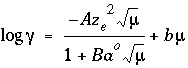, is multiplied times the equivalent fraction to obtain activity for the exchange species. In this equation,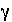is the activity coefficient,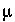is ionic strength, A and B are constants at a given temperature, and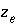is the number of equivalents of exchanger in the exchange species. Optionally, gamma or -g[ amma].

Debye-Hückel a --Parameter a o in the WATEQ activity-coefficient equation.

Debye-Hückel b --Parameter b in the WATEQ activity-coefficient equation.

Line 4: -davies

-davies--Indicates the Davies equation will be used to calculate an activity coefficient. If -gamma or -davies is not input for an exchange species, the activity of the species is equal to its equivalent fraction. If -davies is entered, then an activity coefficient of the form of the Davies equation,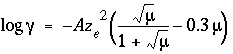, is multiplied times the equivalent fraction to obtain activity for

the exchange species. In this equation,is the activity coefficient,is ionic strength, A is a constant at a given temperature, andis the number of equivalents of exchanger in the exchange species. Optionally, davies or -d[ avies].

### Notes

Lines 1 and 2 may be repeated as necessary to define all of the exchange reactions, with line 1 preceding line 2 for each exchange species. One identity reaction that defines the exchange master species (in example data block, lines 1a and 2a, 1d and 2d) and one reference half-reaction are needed for each exchanger. The identity reaction has a log K of 0.0. The reference half-reaction for each exchanger also will have a log K of 0.0 (in example data block, lines 1b and 2b, 1e and 2e); in the default database file the reference half-reaction is Na + + X - = NaX. Multiple exchangers may be defined simply by defining multiple exchange master species and additional half-reactions involving these master species, as in this example data block.

The theory for activities of exchange species is not well developed. In PHREEQC, the activity of an exchange species is by default assumed to be equal to the equivalent fraction of the species relative to the total equivalents of exchanger. The -gamma identifier allows the equivalent fraction to be multiplied by an activity coefficient to obtain the activity of an exchange species. This activity coefficient is identical to the activity coefficient for an aqueous species calculated by using the WATEQ Debye-Hückel equation. The Davies equation can be used to calculate the activity coefficient of the exchange species by specifying the -davies identifier. The use of these equations is strictly empirical and is motivated by the observation that these activity corrections provide a better fit to some experimental data.

Temperature dependence of log K can be defined with the standard enthalpy of reaction (identifier -delta_h) using the van't Hoff equation or with an analytical expression ( -analytical_expression). See SOLUTION_SPECIES or PHASES for examples.

The identifier -no_check can be used to disable checking charge and elemental balances (see SOLUTION_SPECIES). The use of -no_check is not recommended. By default, the equation given for the exchange species (line 1) is used to determine the mass-action equation and the contribution of the species to each mole-balance equation. Alternatively, the contribution of the species to each mole-balance equation can be defined using the -mole_balance identifier. See SOLUTION_SPECIES and SURFACE_SPECIES for an example. If the -no_check identifier is used, then the -mole_balance identifier is required.

### Example problems

The keyword EXCHANGE_SPECIES is used in example problems 12, 13, and 18. See also listing of default database file in Attachment B for examples.

### Related keywords

EXCHANGE, EXCHANGE_MASTER_SPECIES, SAVE exchange, and USE exchange.

| Next|| Previous || Top |